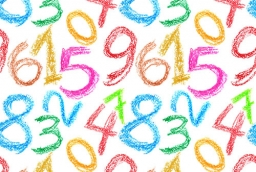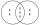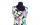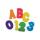# Find unknown number

What is the number between 50 and 55 that is divisible by 2,3,6,9?

n =  54

### Step-by-step explanation:Did you find an error or inaccuracy? Feel free to write us. Thank you!Tips to related online calculators
Do you want to calculate least common multiple two or more numbers?
Do you want to perform natural numbers division - find the quotient and remainder?

## Related math problems and questions:

• Lcm 2Create the smallest possible number that is divisible by numbers 5,8,9,4,3
• LCMWhat is the least common multiple of 5, 50, 14?
• Counting numberWhat is the smallest counting number divisible by 2,5,7,8 and 15?
• LCM of two numberFind the smallest multiple of 63 and 147
• LCD 2The least common denominator of 2/5, 1/2, and 3/4
• Unknown integerFind the smallest integer: divided by 2, the remainder is 1. divided by 3, the remainder is 2. divided by 4, the remainder is 3. ... divided by eight, the remainder is 7, divided by 9 the remainder is 8.
• LCMCommon multiple of three numbers is 3276. One number is in this number 63 times, second 7 times, third 9 times. What are the numbers?
• Divisible by nineHow many three-digit natural numbers in total are divisible without a remainder by the number 9?
• Lcm of three numbersWhat is the Lcm of 120 15 and 5
• Lcm simpleFind least common multiple of this two numbers: 140 175.
• By sixFrom the digits 1,2,3,4 we create the long integer number 123412341234. .. .. , which will have 962 digits. Is this number divisible by 6?
• Tailor masterThere are less than 50m of textile in the tailoring workshop. When cutting on a blouse (consumption 1.5m), no textile is left. When using a cloth (consumption of 3.2m), no textile is left. How many meters of textile are in a tailor's workshop?
• The smallest numberWhat is the smallest number that can be divided by both 5 and 7
• Square roomWhat is the size of the smallest square room, which can pave with tiles with dimensions 55 cm and 45 cm? How many such tiles is needed?
• A number 5A number is divisible by 24, 25, and 120 if it is increased by 20. Find the number.
• The classroomThe classroom is 9 meters long. The width of the classroom is smaller and can be passed in equally long steps of 55 CM or 70 CM. Determine the width of the classroom.
• Divisibility by 12Replace the letters A and B by digits so that the resulting number x is divisible by twelve /find all options/. x = 2A3B How many are the overall solutions?